intenance Management, Dynamic Control1海军92730部队，海南 三亚

2海军工程大学，动力工程学院，湖北 武汉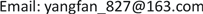1. 引言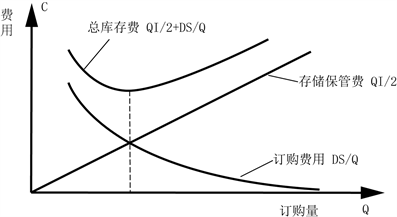Figure 1. Optimal order quantity of the model

2. 库存问题的提出

3. 稳态库存量控制问题Figure 2. Minimum order stock model without safety stock

1) 最佳订货量为 ${Q}^{\ast }=\sqrt{\frac{2DS}{1}}$ (件)

2) 最佳订购次数 ${n}^{\ast }=\frac{D}{{Q}^{*}}=\sqrt{\frac{DI}{2S}}$

4. 动态库存常规控制问题——控制论的应用

4.1. 动态管理决策过程的数学模型建立

${Y}_{t}={f}_{1}\left({Y}_{t-1}\cdots {Y}_{t-i}\cdot {X}_{t}\cdots {X}_{t-j}\right)\text{\hspace{0.17em}}\text{\hspace{0.17em}}\text{\hspace{0.17em}}\left(i\ge 0,\text{\hspace{0.17em}}j\le t\right)$ (1)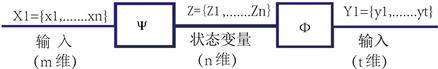Figure 3. Representation of the state space

$\left\{\begin{array}{l}{z}_{t+1}=\psi \left({z}_{t}\cdot {x}_{t}\right)\\ {Y}_{t}=\varphi \left({z}_{t}\cdot {x}_{t}\right)\end{array}$ (2)

$\frac{\text{d}I\left(t\right)}{\text{d}t}=p\left(t\right)-s\left(t\right)$ (3)

$I\left(K+1\right)=I\left(K\right)+P\left(K\right)-S\left(K\right)$ (3)1

4.2. 求解订购–库存模型的方法

$\left\{\begin{array}{l}\frac{\text{d}I\left(t\right)}{\text{d}t}=p\left(t\right)-s\left(t\right)\\ I\left({t}_{o}\right)={I}_{O}\left(初始库存量\right)\end{array}$ (4)

① 订购率P(t)，使订购费用C(p,t)和储存费用H(I,t)总和为最小。

② 求订购率P(t)，使它接近于理想的订购率Pd，即：使库存量I(t)尽量接近理想库存量Id。当系统处于理想稳定状态时，有：

$\left\{\begin{array}{l}p\left(t\right)=pd\\ I\left(t\right)=Id\end{array}$ 成立

${J}_{2}={\int }_{{t}_{o}}^{{t}_{f}}\left[h{\left(I\left(t\right)-Id\right)}^{2}+c{\left(p\left(t\right)-pd\right)}^{2}\right]\text{d}t$ 最小 (5)

$\begin{array}{c}{u}^{*}\left(t\right)=p\left(t\right)-pd=-\frac{p}{20}\cdot I\left(t\right)+\frac{1}{2c}\xi \left(t\right)\\ =\frac{1}{2c}\xi \left(t\right)-K\cdot th\left[K\left({t}_{f}-t\right)\right]\cdot I\left(t\right)\end{array}$

$P\left(t\right)=\frac{1}{2c}\xi \left(t\right)-K\cdot th\left[k\left({t}_{f}-t\right)\right]\cdot I\left(t\right)+pd$Table 1. Quarterly demand for a unit

$Z\left(K+1\right)=Z\left(K\right)+X\left(K\right)-S\left(K\right),\text{\hspace{0.17em}}\text{\hspace{0.17em}}K=1,2,3,4$

${J}_{\mathrm{min}}={\sum }_{k=1}^{4}\left[a{x}^{2}\left(k\right)+bz\left(k\right)\right]$，a，b为加权系数

$\begin{array}{c}{J}_{N}^{*}\left[Z\left(O\right)\right]=\underset{X\left(O\right),\cdots ,X\left(N-1\right)}{\mathrm{min}}{J}_{N}\left[Z\left(O\right),X\left(O\right),\cdots ,X\left(N-1\right)\right]\\ =\underset{X\left(O\right)}{\mathrm{min}}\left\{L\left[Z\left(O\right),X\left(O\right)\right]+{J}_{N}^{*}-1\left[Z\left(1\right)\right]\right\}\end{array}$ (6)

$\begin{array}{l}\therefore {J}_{1}^{*}\left[Z\left(4\right)\right]=0.005{\left[1200-Z\left(4\right)\right]}^{2}+Z\left(4\right)\\ \text{\hspace{0.17em}}\text{\hspace{0.17em}}\text{\hspace{0.17em}}\text{\hspace{0.17em}}\text{\hspace{0.17em}}\text{\hspace{0.17em}}\text{\hspace{0.17em}}\text{\hspace{0.17em}}\text{\hspace{0.17em}}\text{\hspace{0.17em}}\text{\hspace{0.17em}}\text{\hspace{0.17em}}\text{\hspace{0.17em}}\text{\hspace{0.17em}}\text{\hspace{0.17em}}\text{\hspace{0.17em}}\text{\hspace{0.17em}}=0.005{Z}^{2}\left(4\right)-11\cdot Z\left(4\right)+7200\end{array}$

$\therefore {J}_{2}^{*}\left[Z\left(3\right)\right]=\underset{X\left(3\right)}{\mathrm{min}}\left\{0.005{X}^{2}\left(3\right)+X\left(3\right)+{J}_{1}^{*}\left[Z\left(4\right)\right]\right\}$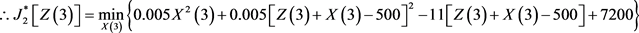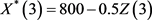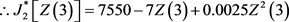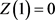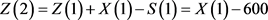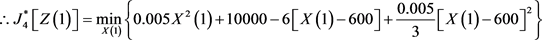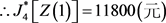Table 2. Cost comparison of different ordering methods

4.3. 订购–库存系统稳定性分析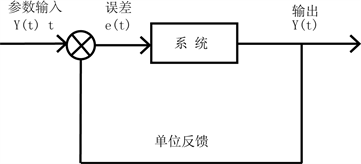Figure 4. Closed-loop system with input size adjusted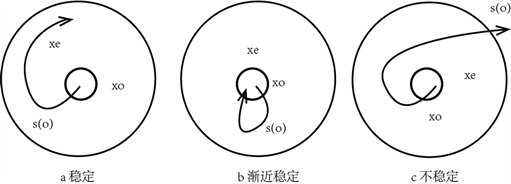Figure 5. Stability of the system

5. 动态库存非常规控制问题——耗散结构理论及其应用

1) 系统处于某种稳定态附近，控制的目的是使系统回到这种稳定态；

2) 对管理系统结构不变，调节之后，也没有出现新的结构，所以系统的数学模型不能改变；

3) 控制和调节的目标已经确定，并在整个过程中保持不变。

1) 非平衡是有序之源。

2) 通过涨落达到有序。

5.1. 熵、有序度、涨落和分支点的讨论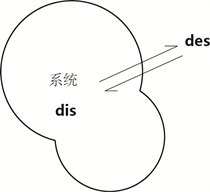Figure 6. Entropy change of non-equilibrium open system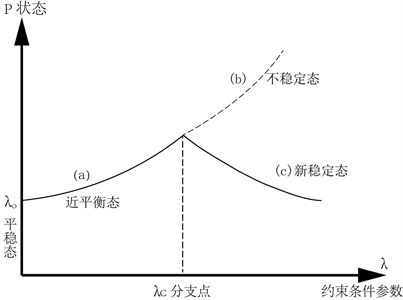Figure 7. Stable way to manage the system

(1)平衡态，系统处于一种稳定状态。对于某一管理系统，输出输入恒定，随时间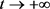，系统宏观状态，不发生任何变化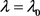5.2. 近平衡态分支过程(a)

5.3. 远离平衡态分支过程(C)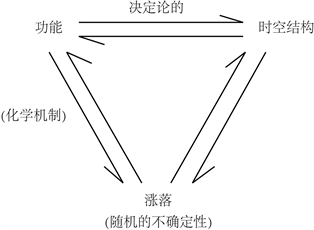Figure 8. Evolution format of dissipative structure self determined sequence

a)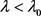稳定态，近平衡区。

b) 不稳定态。

c)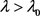新的稳定态出现。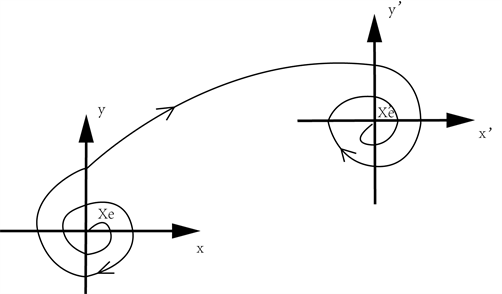Figure 9. Instability of the original structure and stability of the new structure

② 管理系统中，耗散结构模型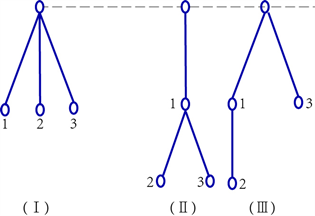Figure 10. Three inventory systems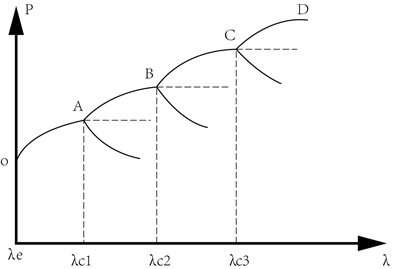Figure 11. Branch diagram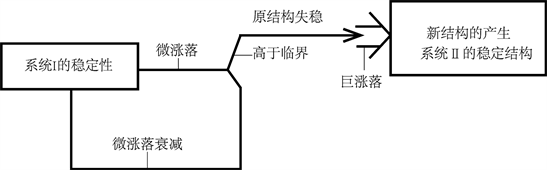Figure 12. Illustrates the evolution from system I to the new system II

6. 结束语

1) 本文探讨了两种新兴科学——现代控制论和耗散结构理论在管理学中的应用。可以看到，它们相互补充、相互完善。现代控制论的方法是一种常规控制，而耗散结构论的自组织控制是一种非常规控制，对任何一个管理系统的控制，或多或少地同时存在，只是人们没有意识到这一点。例如讨论的仓库管理问题总是存在例行的管理，那么现代控制论正适得其所，大有用武之地。

2) 现代控制理论在管理中的应用比较成熟，因此容易做到常规调节的定量化，而耗散结构理论本身还处在探索之中，定性分析多，而定量工作还有待我们进一步开拓。

3) 现代舰船管理工作要求越来越高，本文提出的分析方法在船舶维修管理问题中实际应用还是首次。其他还包括熵、涨落、分支点和耗散结构的讨论，最后给出了一个库存系统的耗散结构模型和应用实例。对突发库存变化类系统来说，由于总有一些转折关头要用到非例行管理方法，这时系统往往面临一个或几个门坎，而自组织控制正好可以用来预测和制定我们的管理策略。

The Application of Cybernetics and Dissipative Structure Theory in Ship Maintenance Inventory Management[J]. 动力系统与控制, 2020, 09(01): 10-21. https://doi.org/10.12677/DSC.2020.91002

1. 1. 浩峰. 普利高津和耗散结构理论[J]. 自然辩证法通讯, 1982(5): 6.

2. 2. 方锦清. 非平衡态研究的最新进展[J]. 自然杂志, 1990(11): 743-751.

3. 3. 王照林. 现代控制理论基础[M]. 北京: 国防工业出版社, 2001.

4. 4. 孙晓静. 飞轮电池控制策略研究及其应用[D]: [博士学位论文]. 兰州: 兰州理工大学, 2013.

5. 5. 付立军, 刘鲁锋, 王刚, 等. 我国舰船中压直流综合电力系统研究进展[J]. 中国舰船研究, 2016, 11(1): 72-79.

6. 6. 刘学, 姜新建, 张超平, 等. 大容量飞轮储能系统优化控制策略[J]. 电工技术学报, 2014, 29(3): 75-82.

7. 7. 陈泽涛, 金朝永. 线性干扰系统的最优解耦控制[J]. 动力系统与控制, 2019, 8(2): 106-113.

8. 8. 贾兴, 孙海义. 复杂网络同步控制方法研究综述[J]. 动力系统与控制, 2018, 7(4): 318-327.

9. 9. 张利, 高存臣, 刘云龙. 时滞广义变结构控制研究新进展[J]. 动力系统与控制, 2018, 7(4): 337-347.

10. 10. 胡凡. 一部有关管理现代化的教材[J]. 自然辩证法通讯, 1981(6): 78.

11. 11. 刘龙英. 动态性经济信息搜集途径刍议[J]. 图书馆论坛, 1999(4): 11-12.